Create a new printable
Answer key also includes questions
Answer key only gives the answers
No answer keyMath Worksheets
Time

Sample - Click above to make a new math worksheet (PDF).
 Name _____________________________Date ___________________
Time
Write the time two ways.

1.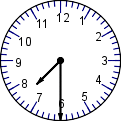half past ______ : ___
2.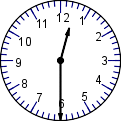half past ______ : ___
3.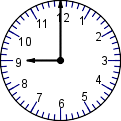___ o'clock___ : ___
4.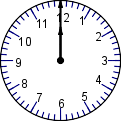___ o'clock___ : ___
 5 * This is a pre-made sheet.Use the link at the top of the page for a printable page.
6.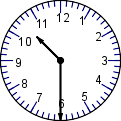half past ______ : ___
7.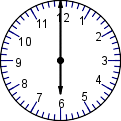___ o'clock___ : ___
8.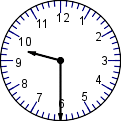half past ______ : ___
9.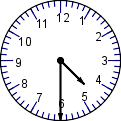half past ______ : ___
10.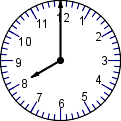___ o'clock___ : ___
11.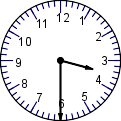half past ______ : ___
12.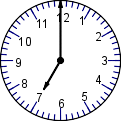___ o'clock___ : ___

 Answer Key

Sample
This is only a sample worksheet.

Create a new printable
Answer key also includes questions
Answer key only gives the answers
No answer keyMath Worksheets
Time